# SAS/IML Time Series Functions and CALL Subroutines

The following functions and CALL subroutines are useful for analyzing and simulating time series data:

Time Series Functions

Function Purpose
ARMASIM simulates an autoregressive moving average (ARMA) series
CONVEXIT computes convexity of a noncontingent cash flow
COVLAG computes autocovariance estimates for a vector time series
DIF computes the difference between a value and a lagged value
DURATION computes modified duration of a noncontingent cash flow
FORWARD computes forward rates
LAG computes lagged values
PV computes the present value
RATES converts interest rates from one base to another
SPOT computes spot rates
YIELD computes yield-to-maturity of a cash-flow stream

Time Series CALL Subroutines

CALL Subroutine Purpose
ARMACOV computes an autocovariance sequence for an ARMA model
ARMALIK computes the log likelihood and residuals for an ARMA model
FARMACOV computes the autocovariance function for an autoregressive fractionally integrated moving average (ARFIMA) model of the form ARFIMA(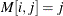)
FARMAFIT estimates the parameters of an ARFIMA () model
FARMALIK computes the log-likelihood function of an ARFIMA() model
FARMASIM generates an ARFIMA () process
FDIF computes a fractionally differenced process
KALCVF computes the one-step prediction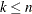and the filtered estimate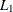, in addition to their covariance matrices. The call uses forward recursions, and you can also use it to obtain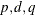-step estimates.
KALCVS uses backward recursions to compute the smoothed estimate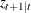and its covariance matrix,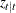, where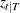is the number of observations in the complete data set
KALDFF computes the one-step forecast of state vectors in a state space model (SSM) by using the diffuse Kalman filter. The call estimates the conditional expectation of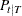, and it also estimates the initial random vector,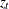, and its covariance matrix.
KALDFS computes the smoothed state vector and its mean squares error matrix from the one-step forecast and mean squares error matrix computed by the KALDFF subroutine
TSBAYSEA performs Bayesian seasonal adjustment modeling
TSDECOMP analyzes nonstationary time series by using smoothness priors modeling
TSMLOCAR analyzes nonstationary or locally stationary time series by using a method that minimizes Akaikes information criterion (AIC)
TSMLOMAR analyzes nonstationary or locally stationary multivariate time series by using a method that minimizes the AIC
TSMULMAR estimates vector autoregressive (VAR) processes by minimizing the AIC
TSPEARS analyzes periodic autoregressive (AR) models by minimizing the AIC
TSPRED provides predicted values of univariate and multivariate ARMA processes when the ARMA coefficients are given
TSROOT computes AR and moving average (MA) coefficients from the characteristic roots of the model, or computes the characteristic roots of the model from the AR and MA coefficients
TSTVCAR analyzes time series that are nonstationary in the covariance function
TSUNIMAR determines the order of an AR process by minimizing the AIC, and estimates the AR coefficients
VARMACOV computes the theoretical cross-covariance matrices for a stationary vector autoregressive moving average (VARMA) (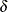) model
VARMALIK computes the log-likelihood function for a VARMA () model
VARMASIM generates a VARMA () time series
VNORMAL generates multivariate normal random series
VTSROOT computes the characteristic roots for a VARMA () model